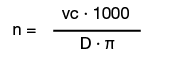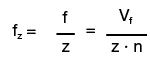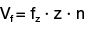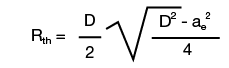Description Formula Definition Rotation speedD = Diameter f = Feed rate fz= Tooth feed rate n = Rotation speed vc = Cutting speed vf = Feed rate speed z = Number of teeth V = 3,14159... Cutting speedFeed rate per toothFeed rate per rotationFeed rate speed## Rth calculation milling

 Symbol Description Metric Formula Rth Roughness depth mm